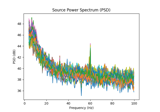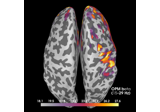# mne.minimum_norm.compute_source_psd¶

mne.minimum_norm.compute_source_psd(raw, inverse_operator, lambda2=0.1111111111111111, method='dSPM', tmin=0.0, tmax=None, fmin=0.0, fmax=200.0, n_fft=2048, overlap=0.5, pick_ori=None, label=None, nave=1, pca=True, prepared=False, method_params=None, inv_split=None, bandwidth='hann', adaptive=False, low_bias=False, n_jobs=1, return_sensor=False, dB=False, verbose=None)[source]

Compute source power spectral density (PSD).

Parameters
rawinstance of `Raw`

The raw data.

inverse_operatorinstance of `InverseOperator`

The inverse operator.

lambda2`float`

The regularization parameter.

method“MNE” | “dSPM” | “sLORETA”

Use minimum norm, dSPM (default), sLORETA, or eLORETA.

tmin`float`

The beginning of the time interval of interest (in seconds). Use 0. for the beginning of the file.

tmax

The end of the time interval of interest (in seconds). If None stop at the end of the file.

fmin`float`

The lower frequency of interest.

fmax`float`

The upper frequency of interest.

n_fft`int`

Window size for the FFT. Should be a power of 2.

overlap`float`

The overlap fraction between windows. Should be between 0 and 1. 0 means no overlap.

pick_ori`None` | “normal”

If “normal”, rather than pooling the orientations by taking the norm, only the radial component is kept. This is only implemented when working with loose orientations.

label`Label`

Restricts the source estimates to a given label.

nave`int`

The number of averages used to scale the noise covariance matrix.

pcabool

If True, the true dimension of data is estimated before running the time-frequency transforms. It reduces the computation times e.g. with a dataset that was maxfiltered (true dim is 64).

preparedbool

If True, do not call `prepare_inverse_operator()`.

method_params

Additional options for eLORETA. See Notes of `apply_inverse()`.

New in version 0.16.

inv_split

Split inverse operator into inv_split parts in order to save memory.

New in version 0.17.

bandwidth

The bandwidth of the multi taper windowing function in Hz. Can also be a string (e.g., ‘hann’) to use a single window.

For backward compatibility, the default is ‘hann’.

New in version 0.17.

Use adaptive weights to combine the tapered spectra into PSD (slow, use n_jobs >> 1 to speed up computation).

New in version 0.17.

low_biasbool

Only use tapers with more than 90% spectral concentration within bandwidth.

New in version 0.17.

n_jobs`int`

The number of jobs to run in parallel (default 1). Requires the joblib package. It is only used if adaptive=True.

New in version 0.17.

return_sensorbool

If True, return the sensor PSDs as an EvokedArray.

New in version 0.17.

dBbool

If True (default False), return output it decibels.

New in version 0.17.

verbose

If not None, override default verbose level (see `mne.verbose()` and Logging documentation for more). If used, it should be passed as a keyword-argument only.

Returns
stc_psd

The PSD of each of the sources.

sensor_psdinstance of `EvokedArray`

The PSD of each sensor. Only returned if `return_sensor` is True.

Notes

Each window is multiplied by a window before processing, so using a non-zero overlap is recommended.

This function is different from `compute_source_psd_epochs()` in that:

1. `bandwidth='hann'` by default, skipping multitaper estimation

2. For convenience it wraps `mne.make_fixed_length_events()` and `mne.Epochs`.

Otherwise the two should produce identical results.

## Examples using `mne.minimum_norm.compute_source_psd`¶